Test: Fourier Analysis of Signals, Energy & Power Signals

# Test: Fourier Analysis of Signals, Energy & Power Signals

Test Description

## 10 Questions MCQ Test GATE ECE (Electronics) 2023 Mock Test Series | Test: Fourier Analysis of Signals, Energy & Power Signals

Test: Fourier Analysis of Signals, Energy & Power Signals for Electronics and Communication Engineering (ECE) 2023 is part of GATE ECE (Electronics) 2023 Mock Test Series preparation. The Test: Fourier Analysis of Signals, Energy & Power Signals questions and answers have been prepared according to the Electronics and Communication Engineering (ECE) exam syllabus.The Test: Fourier Analysis of Signals, Energy & Power Signals MCQs are made for Electronics and Communication Engineering (ECE) 2023 Exam. Find important definitions, questions, notes, meanings, examples, exercises, MCQs and online tests for Test: Fourier Analysis of Signals, Energy & Power Signals below.
Solutions of Test: Fourier Analysis of Signals, Energy & Power Signals questions in English are available as part of our GATE ECE (Electronics) 2023 Mock Test Series for Electronics and Communication Engineering (ECE) & Test: Fourier Analysis of Signals, Energy & Power Signals solutions in Hindi for GATE ECE (Electronics) 2023 Mock Test Series course. Download more important topics, notes, lectures and mock test series for Electronics and Communication Engineering (ECE) Exam by signing up for free. Attempt Test: Fourier Analysis of Signals, Energy & Power Signals | 10 questions in 30 minutes | Mock test for Electronics and Communication Engineering (ECE) preparation | Free important questions MCQ to study GATE ECE (Electronics) 2023 Mock Test Series for Electronics and Communication Engineering (ECE) Exam | Download free PDF with solutions
 1 Crore+ students have signed up on EduRev. Have you?
Test: Fourier Analysis of Signals, Energy & Power Signals - Question 1

### The signals x1(t) and x2(t) are given by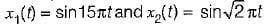If the periods of x1(t) and x2(t) be T1 and T2 respectively, then T1/T2 is equal to

Detailed Solution for Test: Fourier Analysis of Signals, Energy & Power Signals - Question 1

x1(t) = sin15πt is a periodic signal having period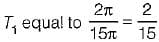x2(t) = sin√2 πt is a periodic signal having period T2 equal to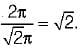∴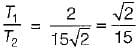Test: Fourier Analysis of Signals, Energy & Power Signals - Question 2

### The Fourier transform of a unit step function is

Detailed Solution for Test: Fourier Analysis of Signals, Energy & Power Signals - Question 2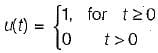∴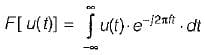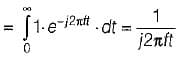Test: Fourier Analysis of Signals, Energy & Power Signals - Question 3

### Assertion (A): A frequency domain representation of a signal shows the frequency content and indicates the shape of the waveform or the combined amplitude of all the input components at any specific time. Reason (R): In frequency domain, a signal is represented by its frequency spectrum.

Detailed Solution for Test: Fourier Analysis of Signals, Energy & Power Signals - Question 3

A frequency domain representation of a signal shows the frequency content but not necessarily indicate the shape of the waveform or the combined amplitude of all the input components at any specific time. Hence, assertion is a false statement. Reason is a true statement because in frequency domain, a signal is represented by its frequency spectrum and to obtain frequency spectrum of a signal, Fourier series and Fourier transform are used.

Test: Fourier Analysis of Signals, Energy & Power Signals - Question 4

Which of the following option is not correct for obtaining the Fourier transform of a signal x(f)7

Detailed Solution for Test: Fourier Analysis of Signals, Energy & Power Signals - Question 4

For obtaining the Fourier transform of a signal x(t), it must have infinite number of discontinuities in any finite interval T.

Test: Fourier Analysis of Signals, Energy & Power Signals - Question 5

Match List-I (Operations on x(t)) with List-ll (X(ω)/Fourier transform) and select the correct answer using the codes given below the lists: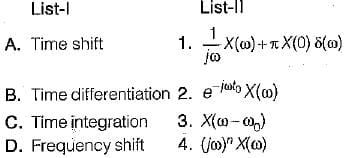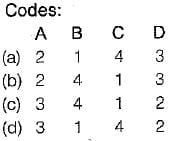Detailed Solution for Test: Fourier Analysis of Signals, Energy & Power Signals - Question 5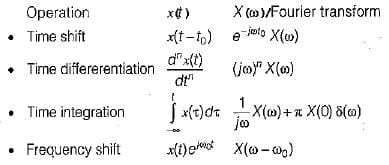Test: Fourier Analysis of Signals, Energy & Power Signals - Question 6

Assertion (A): The Parseval’s theorem implies superposition of the average powers.
Reason (R): The interpretation of the Parseval’s theorem is that the total average power of the signal x(t) can be found by squaring and adding the heights of the amplitude lines in the spectrum of the periodic signal x(t).

Test: Fourier Analysis of Signals, Energy & Power Signals - Question 7

Fourier series analysis is a tool used to
1. analyse any periodic and non-periodic signal.
2. find how many frequency components are present in the signal.
3. find the relative phase difference between various frequency components.
4. represents a waveform in the form of sum of infinite number of exponential terms.
5. find the amplitude of various terms in the series.
Which of the statements given above are correct?

Detailed Solution for Test: Fourier Analysis of Signals, Energy & Power Signals - Question 7

Fourier series analysis is a tool used to
1. analyse any periodic signal only.
2. find how many frequency components are present in the signal.
3. find the relative phase difference between various frequency components.
4. represents a periodic waveform in the form of sum of infinite number of sine and cosine terms.
5. find the amplitude of various frequency components of the series.
Hence, statements 2, 3 and 5 are only correct.

Test: Fourier Analysis of Signals, Energy & Power Signals - Question 8

The power spectral density of a power signal x(t) is shown in figure below.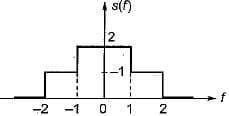The average power of the signal is

Detailed Solution for Test: Fourier Analysis of Signals, Energy & Power Signals - Question 8

The total average power of a signal is the area of curve under s(f).
Hence,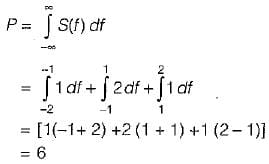Test: Fourier Analysis of Signals, Energy & Power Signals - Question 9

The energy of a causal exponential pulse shown in figure is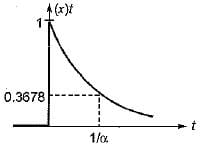Detailed Solution for Test: Fourier Analysis of Signals, Energy & Power Signals - Question 9

Given,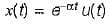∴ Normalized energy of given signal is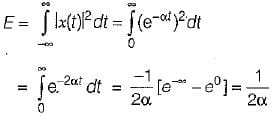Test: Fourier Analysis of Signals, Energy & Power Signals - Question 10

The Fourier transform of the decaying exponential pulse shown in figure is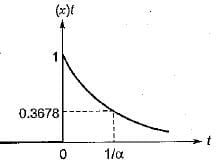Detailed Solution for Test: Fourier Analysis of Signals, Energy & Power Signals - Question 10

The given signal can be represented as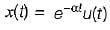(Time constant,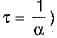∴ Fourier transform of x(t) is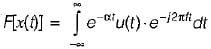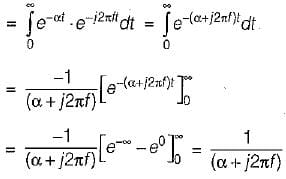## GATE ECE (Electronics) 2023 Mock Test Series

21 docs|263 tests
Information about Test: Fourier Analysis of Signals, Energy & Power Signals Page
In this test you can find the Exam questions for Test: Fourier Analysis of Signals, Energy & Power Signals solved & explained in the simplest way possible. Besides giving Questions and answers for Test: Fourier Analysis of Signals, Energy & Power Signals, EduRev gives you an ample number of Online tests for practice

## GATE ECE (Electronics) 2023 Mock Test Series

21 docs|263 tests(Scan QR code)